# Feed Forward and Back Propagation in a Neural NetworkImage courtsey -https://tenor.com/view/myd-ed-bangers-moving-men-moving-men-gif-19080124

In this write up a technical explanation and functioning of a fully connected neural network which involves bi direction flow, first a forward direction knows as Feed forward and a backward direction known as back propagation.

Take the below example of a fully connected neural network which has two inputs, one hidden layer with 2 neurons and an output layer where 2 neurons represent the two outputs so it can be deemed as a binary class classification.

Hidden layer consists of a summation and an activation function to it, activation functions are used to introduce non linearity other than acting as a filter for neurons to pass and the commonly used activation functions are Relu, Tanh and Sigmoid and Softmax.

A typical neuron cell looks like below. As can be seen the initial weights which may be random gets multiplied by the feature vector and gets added up in a neuron, the activation then decides whether to fire up the neuron or not.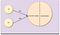A typical neuron with inputs weights and internal assembly containing summation and activation

A fully connected network looks like below where each input is connected to each neuron in the hidden layer by weights associated with each connection. The relation is many to many.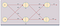A Fully connected NN with one hidden having two neuron along with output layer

Consider a scenario where the problem is a binary class classification and the expected accuracy is.99 and 0.01 resp for both the classes. You can also try with the label outcome as 1 and 0.

let’s have a look below at the assumed values which are required initially for the feed fwd and back prop. The hidden layer activation function is assumed to be sigmoid and the weights are random initially.Weight matrix, weights are randomly initialized

The summation equation

∑h1= X1W1+X2W2 = 0.2*0.15+0.3*0.25 = 0.105 .

∑h2 = X1W3+X2W4= 0.2*0.35+0.3*0.45 = 0.205

These values which are the sum at hidden nodes then go for a non-linear transformation (sigmoid in this case) the sigmoid transformed values are the output of the hidden neurons which is given by.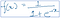Sigmoid expression

After feeding the summed up values ( 0.105 and 0.205) the output of the neuron becomes.

Oh1=0.526

Oh2=0.551

These outputs are further multiplied by the weight matrix for the next layer the equation becomes.

∑OutH1 = Oh1*W5+Oh2*W6 = 0.526*0.55+0.551*0.65 = 0.647

∑OutH2 = Oh1*W7+Oh2*W8 = 0.526*0.75+0.551*0.85 = 0.862

We have taken the sigmoid function at the output node but for in practice Softmax is more appropriate especially when it comes to multiclass classification.

The Output will finally come to

Sigmoid(∑OutH1) and Sigmoid(∑OutH2)

O1= .6563 and O2=0.703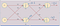Feed Fwd calculation

Model Error during Forward pass

The deviation of the prediction from the actual (sometimes referred as the ground truth) is called as an Error, error at each output node is summed and called as the total error. There are different ways of calculating the error depending on the loss function and in our example chosen the error is taken as.

E= 1/2 (Actual-Predicted)2

E01= ½(.99-.65)2 = 0.0578

E02= ½(.01-.70)2 = 0.238

Thus the total Error done by the model is

E01+E02 =0.0578+0.238

Error =0.295

The main aim of algorithm optimization is to minimize this error and cause the predicted output closer and closer to the actual output. The total error is obtained at the last step and must be communicated backwards to each and every processing neuron (except the input layer) in order to update the Initial random weights.

Once the weights are updated, another round of prediction(Feed fwd) will happen as mentioned in the graph but with a different set of weights and it is expected that the prediction will be closer to the actual output. This process continues till the time when there remains no value in further updating the weights I,e the reduction in the error is not significant.

The distribution of the error is not constant. If we think of the error distribution as “Total Error divided by number of neurons”, we are wrong here. The Error is rather distributed proportionally to each neuron according to the magnitude of the error done by each neuron.

Backpropagation helps in distributing the error to each weight as the contribution of each neuron towards the error is different. Let’s see the constructs and nuances of backpropagation in detail.

Backpropagation.

Backpropagation (BP) is a mechanism by which an error is distributed across the neural network to update the weights, till now this is clear that each weight has different amount of say in the total error hence in order to reduce the total error the weights needs to be updated accordingly, how much each individual weight changes with respect to one unit of change in error is the crux of backprop.

This is also called as the gradient, so finding gradient wrt total error for each weight is what Backprop does.

Mathematically gradient is expressed as partial derivation or differentiation.

Let’s take one of the weights as an example and see how to understand the change in weight wrt change in the error.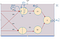Backprop representation

The total Error term E does not relate to any of the weight W directly and hence in other words if we want to calculate the change in W5 wrt E we have to decode sequences in reverse order.

The Change in Weight W5 depends on how the summation changes wrt activation which in turn depends on how activation changes wrt Error done by the first output neuron which in turn depends on the total Error , so now expressing mathematically the gradients, the gradient of w5 wrt total Error will unfold to.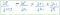Chain rule of partial derivation

The right-hand Side of the equation which expands the dimensions on how W5 id related to E unfolds a chain and hence known as “The Chain Rule” so that’s why in technical parlance it is said that in a Neural Network backpropagation happens through the Chain rule.

Updation of weights.

Let’s consolidate all the numbers here once again to understand the gradient calculations.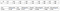Matrix after the feed forward loop

Lets see how W5 would be adjusted by backpropagation of the total error, Since W5 is not directly connected to the total Error we have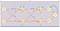Back Prop representation

1. Gradient of total error wrt O1.

The first gradient that would be calculated, will be how much the total error changes wrt change in the outputs (remember for a binary class we have two predictions and hence two outputs).

The error calculation depends on the loss function that is defined for the neural network and in our case the loss function is assumed to be squared error function and hence the error formula would be ½(actual-target)2 .

While calculating the derivative wrt E1 , the error term E2 will be constant and the derivative wrt E2 will be zero hence the derivative becomes.

E1= ½(O1-actual1)2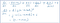Partial derivation of Error wrt Output

(O1-Actual1) = (0.647–1) = -0.353

Similarly, for the change in error wrt change on output2

E2= ½ (O2 -actual2)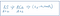partial derivation wrt to output2

(O2 -actual2) = (0.862–0.01) = 0.852

2. Gradient of O1 wrt h1.

Now that we have seen how the error changes wrt input, the next gradient to note would be how much the output (O1 and O2 resp) changes with respect to the total sum of inputs (∑OutH1 and ∑OutH2), if you see the above FC network, now much activation function changes with respect to sigma.

The activation function chosen here is sigmoid and can be expressed as.

∑OutH1 = 1/ (1 + e o1)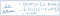partial derivation of sigmoid expression (activation function)

3. Gradient of ∑OutH1 wrt w5.

Finally we need to see how W5 changes wrt change in the net summation (∑OutH1) to understand that lets understand the relation first.

∑OutH1= Oh1w5 + Oh2w6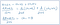Partial derivative of net input wrt weight(w5)

While deriving change in the net sum wrt w5 , only w5 will be treated as a variable keeping w6 constant and hence the derivative Oh2W6 wrt w5 becomes zero. So the result comes as Oh1

Oh1 => 0.526

Since we have reached to the chain below, lets rewrite the chains.

->Change in Error wrt prediction

-> Change in output neuron(activation) wrt net input.

->Change in net input wrt weight

So to get the change in the error wrt W5 can be written as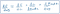Chain rule of showing partial derivation

The total product comes up (-0.34*0.224*0.526)= -0.04

If you think that this is the value by which weight W5 will be adjusted but not actually! The gradient calculated for W5 wrt total Error will be multiplied by a factor which can vary from 0 to 1 known as “Learning Rate”(often denoted by Eta (ⴄ)) of the model ( hyper parameter), let’s assume the rate as 0.3 for now, so the updated weight becomes.

New weight = Initial weight — adjusted weight.

adjusted weight = learning rate * rate of change of weight wrt change in error.

putting all these together.

W5 = W5-ⴄdE/dW5

= 0.55 -0.5*(-0.04)

= 0.57

So this would be the value of new weight W5 and again a feed forward network will fire followed by backprop, this process will continue till we reach no of epochs pre-defined or there is no change in validation accuracy and process can trip early ( known as early stopping).

Conclusion.

· A Neural network is a graph where the inputs are connected to the neurons and if each input is connected to every neuron the assembly is known as fully connected neural network(FCNN).

· A neuron consists of two parts, summation and activation. Summation is the sum of weights multiplied by the feature vector. weights are numerical values initialized randomly.

· Activation function plays a very important role in introducing non linearity and also acts as a gate to let the neurons go or stop them.

· A FCNN can have many hidden layers and each hidden layer can have many neurons which is defined while coding the structure for FCNN.

· The output layer will do the prediction and the predicted values are compared against the actual values(ground truth), the difference between the actual and the predicted is calculated .

· A loss function is defined to calculate and expressed the error done as a form of loss.

· The aim is to minimize the loss (error) and to change the randomly initialized weights to a value which helps to give better predictions.

· Backpropagation is used to adjust the weight systematically by factoring how much each weight must change wrt the total error.

· The feed forward and back propagation continues until the error is minimized or epochs are reached.

Please read more about the hyperparameters, and different type of cost (loss) optimization functions

# Happy learning to one and all!

Deep learning architect| Lifelong Learner|

## More from Saurabh Kirar

Deep learning architect| Lifelong Learner|